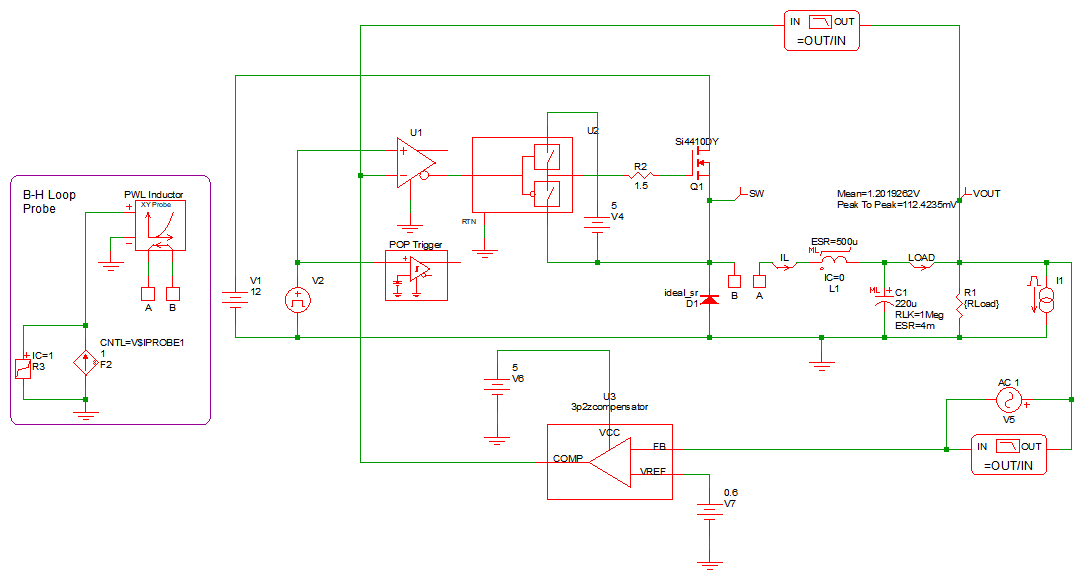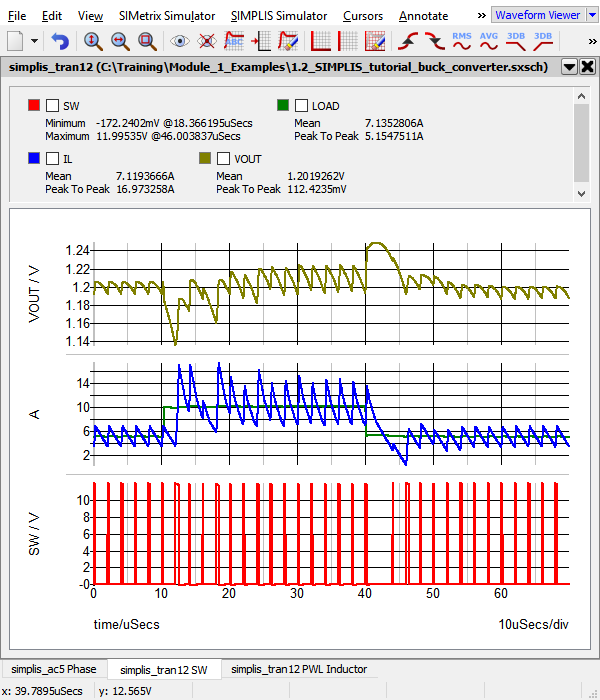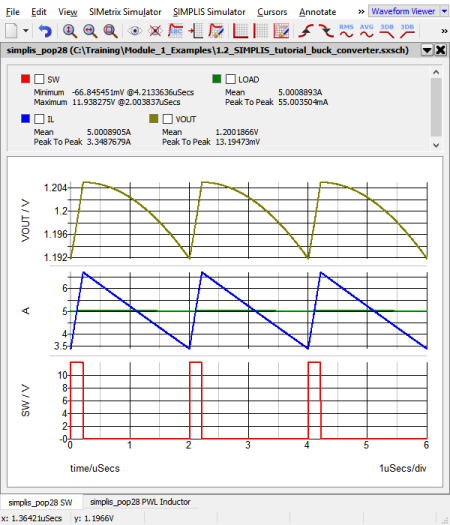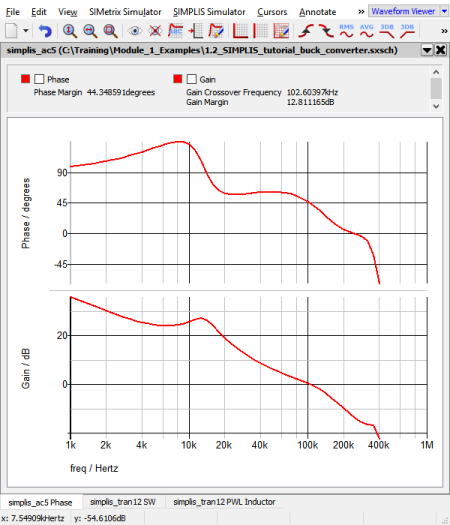# 1.0.1 SIMPLIS is a Time-Domain Simulator, all the Time, for Every Analysis, Period

SIMPLIS is a time-domain simulator optimized for switching power converters. Most users come to SIMPLIS with some experience using SPICE simulators, which work on an entirely different set of principles. This topic introduces an important difference between SIMPLIS and SPICE. SIMPLIS is exclusively a time-domain simulator, even though it can present its time-domain results very accurately in frequency-domain plots.

In this topic:

## Key Concepts

This topic addresses the following key concepts:

• The SIMPLIS simulator is a time-domain simulator which uses Piecewise Linear (PWL) models.
• The AC Analysis requires a periodic operating point (POP) analysis to be run first to find the switching steady-state operating point.
• The Transient analysis run after a POP analysis is initialized to the POP steady-state operating point.

## What You Will Learn

In this topic, you will learn the following:

How SIMPLIS analyzes circuits exclusively in the time domain. This includes the SIMPLIS AC analysis, which is carried out in the time domain.

## Getting Started: Running SIMPLIS

In this exercise, you will simulate a synchronous buck converter in the each of the three SIMPLIS analyses, Periodic Operating Point, AC analysis and Transient Analysis.

1. Open the schematic titled 1.2_SIMPLIS_tutorial_buck_converter.sxsch.
Result: The buck converter schematic opens:2. To simulate the design, press F9 or from the menu bar, select Simulator > Run Schematic .
Result:
• The SIMPLIS simulator simulates the same time-domain nonlinear schematic in each of the three analysis modes, Periodic Operating Point (POP), AC, and Transient.
• The SIMPLIS Status window opens when the simulation is first launched, and the waveform viewer displays the simulation results as the simulation progresses.
• The results from the POP analysis are not displayed, as the transient analysis was specified. The transient simulation begins at the operating point found by the POP analysis and only the transient analysis results are displayed.
• The simulation results displayed in the waveform viewer include waveforms plotted versus time as well as time-domain waveforms that are plotted against each other using X-Y plots, where time in an implicit variable.After running the simulation, the waveform viewer contains a number of graphs. The left-most tab has the gain and phase of the converter control loop taken from the AC Analysis. The other tabs have the results of the transient analysis.

## Discussion

SIMPLIS runs these three analyses in the following order:

1. Periodic Operating Point (POP) Analysis
2. AC Analysis
3. Transient Analysis
The first analysis run is the POP analysis. The POP analysis finds the switching steady-state operating point of the circuit. This steady-state operating point is then used to:
1. Perform a small signal AC analysis on the circuit at this steady-state operating point.
2. Initialize the circuit for the following transient analysis.

Each of these analyses are executed in the time domain, which is exactly what happens on the lab bench. The Periodic Operating Point analysis is discussed in detail in section 1.0.5 POP and AC Analysis, for now think of the POP analysis as a way to accelerate the process of getting to steady state. A key point to remember is that without the Periodic Operating Point, you cannot run an AC analysis on the circuit.

### How the SIMPLIS AC Analysis works

When you go into the lab and connect your switching power system to a network analyzer, you are measuring the AC response of the circuit in the time domain. Your circuit doesn't suddenly change to a averaged small signal model when the network analyzer is connected to it. The SIMPLIS AC analysis works exactly in the same way; it performs an AC analysis on a time-domain simulation model:

1. You run a POP analysis on the circuit. This analysis finds the large signal steady state operating point of the circuit.
2. You run an AC analysis on the circuit using the POP analysis as the operating point. For each frequency in the sweep, the AC analysis does the following:
1. Sets all AC sources (for AC analysis) to a common frequency - the analysis, or perturbation, frequency. The analysis frequency is not the switching frequency where the POP analysis took place - it is the frequency where the small signal analysis is run.
2. These AC sources are time-domain sinusoidal, and the amplitudes of each source is set to an infinitesimally small number.
3. SIMPLIS simulates the time domain response of the circuit to the AC perturbation.
4. Using Fourier methods, SIMPLIS extracts the small signal response of the circuit from the time-domain data.

During an AC sweep, steps a-d above are repeated for each frequency in the sweep. The circuit is perturbed by a single frequency, and that frequency is stepped.

The time domain POP waveforms and the frequency-domain loop response of the Synchronous Buck Converter are shown below. The frequency response of the circuit is valid at the steady-state condition found during the POP analysis.

 Time Domain Waveforms Frequency Response of Time Domain ModelThe transient analysis is similar to a transient analysis in other simulators, except it typically runs much faster.

## Conclusions and Key Points to Remember

SIMPLIS operates just like your circuit in the laboratory - in the time domain.

• Your power electronic switching system in the lab has no concept of a DC operating point or an AC small signal model.
• The power switches turn ON and then OFF as determined by a modulator control circuit that senses the output and tries to regulate the circuit performance accordingly.
• If the circuit is not switching, it is not working correctly.
• A switching converter has no DC operating point.
• An averaged AC model is a theoretical construct, it does not exist on the lab bench.
• If you cannot successfully perform a POP analysis on your circuit:
1. You cannot easily evaluate the AC performance of the circuit. (Caveat: DVM has a Multi-Tone AC analysis, but this takes much longer than the combination of a POP and AC analysis.)
2. In the vast majority of cases, your simulations will take much longer, since you will have to first wait for the converter to reach steady state before you can perform your intended analysis.